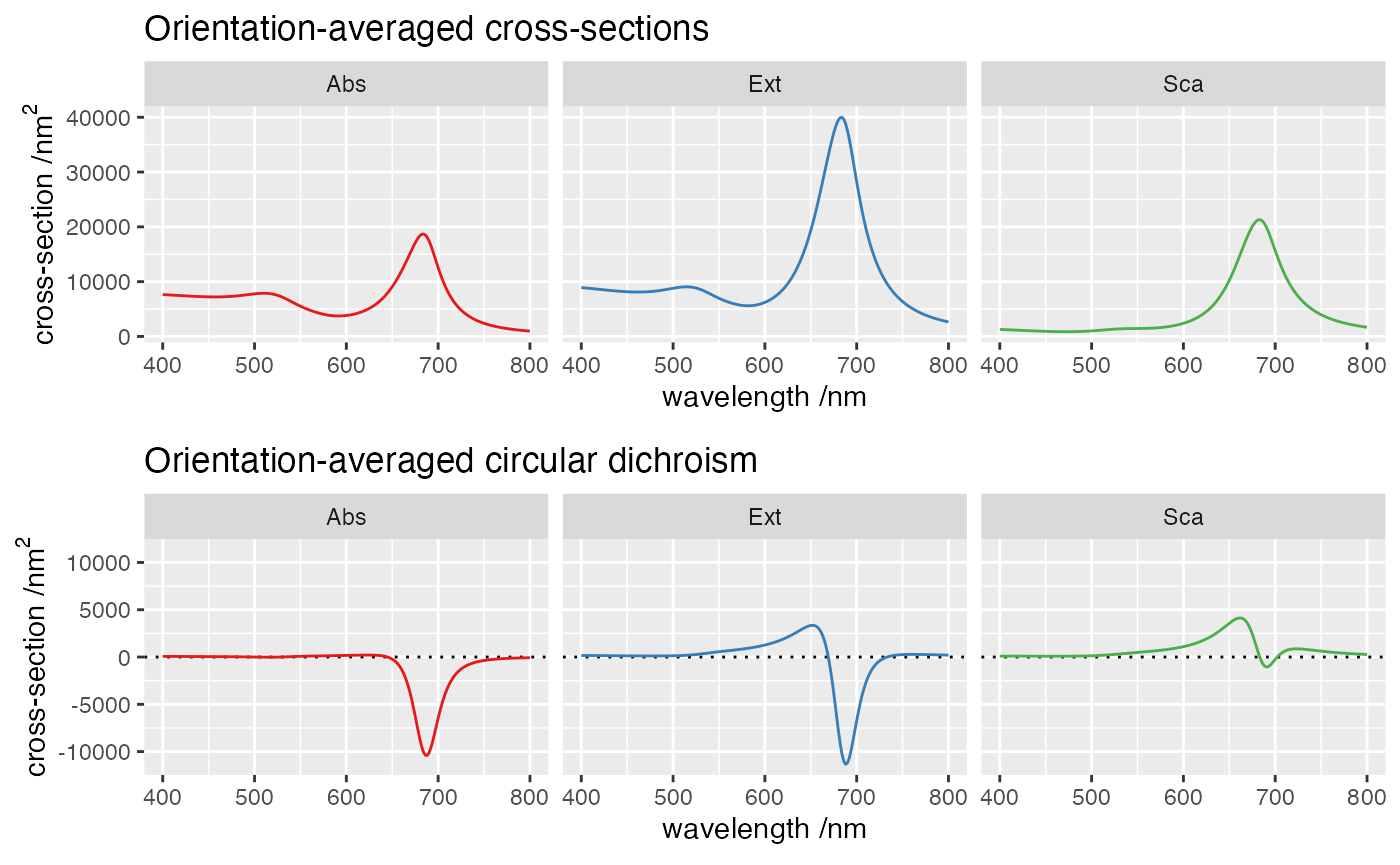## Objective

This example illustrates the calculation of far-field spectra for a dimer of particles described by external T-matrix files. The structure consists of two prolate gold spheroids in water, oriented in a chiral “fingers crossed” configuration that yields strong optical activity.

This simulation uses the following input file

ModeAndScheme 2 3
MultipoleCutoff 5
Wavelength 400 800 200
Medium 1.7689 # careful: be consistent with external T-matrix

TmatrixFiles 1
"tmat_Au20x50_Nmax4.tmat"

OutputFormat HDF5 cross_sections

# dimer, dihedral pi/4
Scatterers 2
TF1 0 -50 0.0 50 0.0 0.0 0.0  2.5
TF1 0  50 0.0 50 0.0 0.7853982 0  2.5

The T-matrix file was previously created with SMARTIES using these parameters

head -n14 smarties.m
clearvars

wavelength = 400:2:800;
epsilon=epsAu(wavelength);
medium=1.33;

% stParams.a=20; stParams.c=50;
stParams.a=10; stParams.c=20;
stParams.N=4; stParams.nNbTheta=40;

stOptions.bGetR = false;
stOptions.Delta = 0;
stOptions.NB = 0;
stOptions.bGetSymmetricT = false;

The command to run the example is simply

../../build/terms input > log

The full log contains basic details of the calculations, and finishes with the timing.

Show log file (click to open)
readInputFile> Parsing file input

mode=2 => spectrum_FF for far-field quantities
scheme=3 => Seek T^(j) using Mackowski's approach
Supplied ncut(1)=  5
Setting ncut(2)= ncut(1)
Setting ncut(3)=  -8
Wavelength LB (nm):   400.000000
Wavelength UB (nm):   800.000000
nsteps, step:       200    2.0000
Constant host epsilon=  1.7689E+0
OutputFormat=HDF5
All output files are stored in file "cross_sections.h5                                               "

with nscat=    2

scatID String                  x          y          z         R_0
1   TF1                 0.0000E+0 -5.0000E+1  0.0000E+0  5.0000E+1
2   TF1                 0.0000E+0  5.0000E+1  0.0000E+0  5.0000E+1

scatID Details
1   Euler angles:  0.0000000  0.0000000  0.0000000 asp. ratio:  2.5000000
2   Euler angles:  0.0000000  0.7853982  0.0000000 asp. ratio:  2.5000000

spectrumFF> ===== Wavelength:   400.00 (nm) ======================

solve> Prestaging...
solve> Staging and solving/inverting...
solve> Done!

diagnoseTmat> WARNING: Tr(Tmat) not converged to 1.0E-08 for n <= nmax=  5

diagnoseTmat> WARNING: Max(|SymErr(Tmat)|)/Max(|Tmat|)= 5.7E-04

calcOAprops> <csExt>=  8.92356552E+03 nConv=  0
<csSca>=  1.28057254E+03 nConv=  0
<csAbs>=  7.64299298E+03

<cdExt>=  1.59634366E+02
<cdSca>=  9.09944193E+01
<cdAbs>=  6.86399471E+01

calcCs> csExt=  8.11985185E+03 nConv=  0
csSca=  1.11248256E+03 nConv=  0
csAbs=  7.00736929E+03

...
termsProgram> Program run time (CPU & real in s):  1.049E+00  8.280E-01

## Orientation-averaged results

Rows: 3,618
Columns: 5
$wavelength <dbl> 400, 400, 400, 400, 400, 400, 402, 402, 402, 402, 402, 402,…$ crosstype  <chr> "Abs", "Abs", "Abs", "Abs", "Abs", "Abs", "Abs", "Abs", "Ab…
$variable <chr> "total", "I1", "I2", "I3", "I4", "I5", "total", "I1", "I2",…$ dichroism  <dbl> 6.863995e+01, 6.016556e+01, 9.546410e+00, -1.094083e+00, 2.…
\$ average    <dbl> 7642.9929773, 5955.4637817, 1558.6333188, 123.3448785, 5.41…This chiral dimer produces strong circular dichroism.

Last run: 23 February, 2022